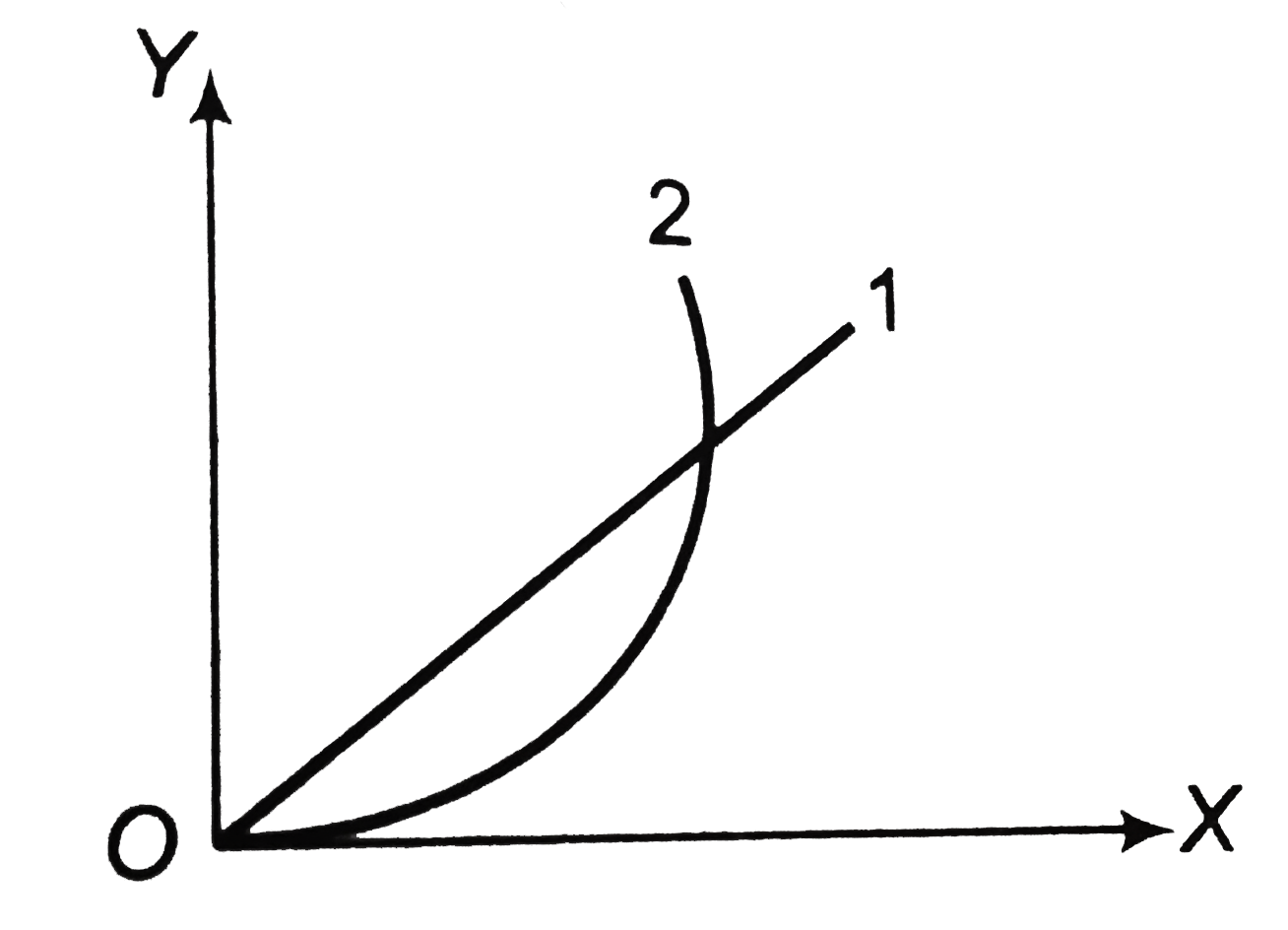# A particle is resting over a smooth horizontal floor. At t = 0, a horizontal force starts acting on it. Magnitude of the force increases with time a

44 views
in Physics
closed
A particle is resting over a smooth horizontal floor. At t = 0, a horizontal force starts acting on it. Magnitude of the force increases with time according to law F = alpha t, Where alpha is a positive constant. From figure, which of the following statements are correct ?A. Curve 1 shows acceleration against time
B. Curve 2 shows velocity against time
C. Curve 2 shows velocity against acceleration
D. none of these

by (84.1k points)
selected

F=alphat
ma=alphat rArr aalphat
curve 1 is against time (mdv)/(dt) = prop t.
Integrating this we get v prop t this implise parabola.
also v prop a^(2)rArr  curve 3 shows velocity against acceleration.